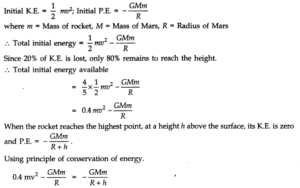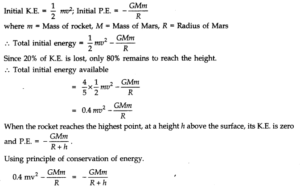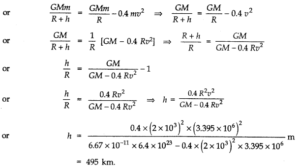# Chapter 8 – Gravitation Questions and Answers: NCERT Solutions for Class 11 Physics

Class 11 Physics NCERT book solutions for Chapter 8 - Gravitation Questions and Answers.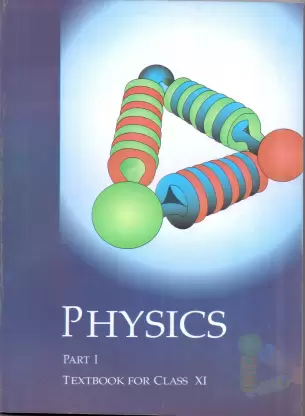## Question 8.3. Suppose there existed a planet that went around the Sun twice as fast as the Earth. What would be its orbital size as compared to that of the Earth?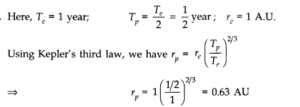## Question 8.4. Io, one of the satellites of Jupiter, has an orbital period of 1.769 days and the radius of the orbit is 4.22 x 108m. Show that the mass of Jupiter is about one-thousandth that of the Sun.

### Answer.For a satellite of Jupiter, orbital period, T1= 1.769 days = 1.769 x 24 x 60 x 60 s Radius of the orbit of satellite, r1= 4.22 x 108m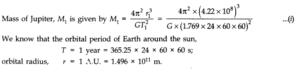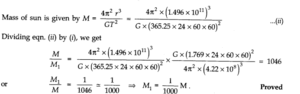## Question 8.5. Let us assume that our galaxy consists of 2.5 x 1011stars each of one solar mass. How long will a star at a distance of 50,000 ly from the galactic centre take to complete one revolution? Take the diameter of the Milky way to be 105ly.

### Answer:Here, r = 50000 ly = 50000 x 9.46 x 1015m = 4.73 x 1020m M = 2.5 x 1011solar mass = 2.5 x 1011x (2 x 1030) kg = 5.0 x 1041kg We know that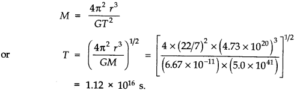## Question 8.7. Does the escape speed of a body from the Earth depend on (a) the mass of the body, (b) the location from where it is projected, (c) the direction of projection, (d) the height of the location from where the body is launched?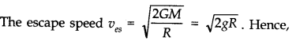## Question 8. 10. In the following two exercises, choose the correct answer from among the given ones: The gravitational intensity at the centre of a hemispherical shell of uniform mass density has the direction indicated by the arrow (see Fig.) (i) a, (ii) b, (iii) c, (iv) 0.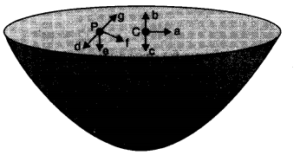## Question 8. 12. A rocket is fired from the earth towards the sun. At what distance from the earth’s centre is the gravitational force on the rocket zero? Mass of the sun = 2 x 1030kg, mass of the earth = 6 x 1024kg. Neglect the effect of other planets etc. (orbital radius = 1.5 x 1011m).

### Answer:Mass of Sun, M = 2 x 1030kg; Mass of Earth, m = 6 x 1024kg Distance between Sim and Earth, r = 1.5 x 1011m Let at the point P, the gravitational force on the rocket due to Earth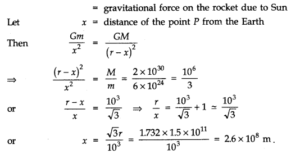## Question 8. 13. How will you ‘weigh the sun’, that is, estimate its mass? The mean orbital radius of the earth around the sun is 1.5 x 108km.

### Answer:The mean orbital radius of the Earth around the Sun R = 1.5 x108km = 1.5 x 1011m Time period, T = 365.25 x 24 x 60 x 60 s Let the mass of the Sun be M and that of Earth be m.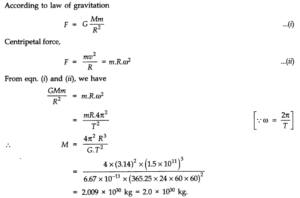## Question 8. 14. A Saturn year is 29.5 times the Earth year. How far is the Saturn from the Sun if the Earth is 1.50 x 108km away from the Sun?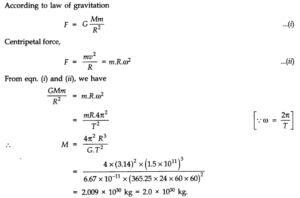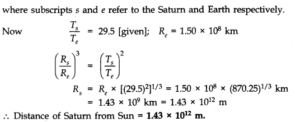## Question 8. 15. A body weighs 63 N on the surface of the Earth. What is the gravitational force on it due to the Earth at a height equal to half the radius of the Earth?

### Answer:Let ghbe the acceleration due to gravity at a height equal to half the radius of the Earth (h = R/2) and g its value on Earth’s surface. Let the body have mass m.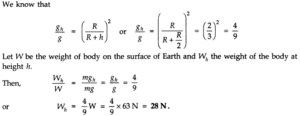## Question 8. 16. Assuming the earth to be a sphere of uniform mass density, how much would a body weigh half way down to the centre of the earth if it weighed 250 N on the surface?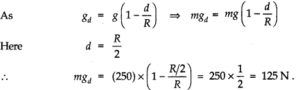## Question 8. 17. A rocket is fired vertically with a speed of 5 km s-1from the earth’s surface. How far from the earth does the rocket go before returning to the earth? Mass of the earth = 6.0 x 1024kg; mean radius of the earth = 6.4 x 106m; G = 6.67 x 10-11N m2kg-2.

### Answer:Initial kinetic energy of rocket = 1/2 mv2= 1/2 x m x (5000)2 = 1.25 x 107mJ At distance r from centre of earth, kinetic energy becomes zero .•. Change in kinetic energy = 1.25 x 107– 0 = 1.25 x 107m J This energy changes into potential energy. Initial potential energy at the surface of earth = GMem/’r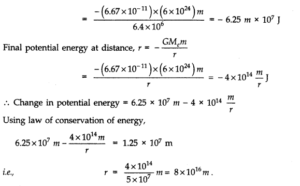## Question 8. 18. The escape speed of a projectile on the Earth’s surface is 11.2 km s-1. A body is projected out with thrice this speed. What is the speed of the body far away from the Earth? Ignore the presence of the Sun and other planets.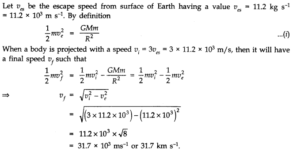## Question 8. 19. A satellite orbits the earth at a height of 400 km above the surface. How much energy must be expended to rocket the satellite out of the earth’s gravitational influence? Mass of the satellite = 200 kg; mass of the earth = 6.0 x1024kg; radius of the earth = 6.4 x106m; G = 6.67 x10-11N m2kg-2.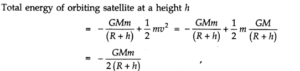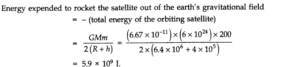## Question 8. 20. Two stars each of one solar mass (=2 x1030kg) are approaching each other for a head on collision.When they are at a distance109km, their speeds are negligible. What is the speed with which they collide? The radius of each star is104km. Assume the stars to remain undistorted until they collide. (Use the known value of G).

### Answer:Here, mass of each star, M = 2 x1030kg Initial potential between two stars, r =109km =1012m. Initial potential energy of the system = -GMm/r Total K.E. of the stars = 1/2Mv2+ 1/2Mv2 where v is the speed of stars with which they collide. When the stars are about to collide, the distance between their centres, r’ = 2 R. :. Final potential energy of two stars = -GMm/2R Since gain in K.E. is at the cost of loss in P.E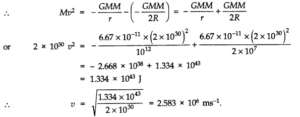## Question 8. 21. Two heavy spheres each of mass 100 kg and radius 0.10 mare placed 1.0 m apart on ahorizontal table. What is the gravitational field and potential at the mid point of the line joining the centres of the spheres ? Is an object placed at that point in equilibrium ? If so, is the equilibrium stable or unstable?

### Answer:Here G = 6.67 x 10-11Nm2kg-2; M = 100 kg; R = 0.1 m, distance between the two spheres, d = 1.0 m Suppose that the distance of either sphere from the mid-point of the line joining their centre is r. Then r=d/2=0.5 m. The gravitational field at the mid-point due to two spheres will be equal and opposite.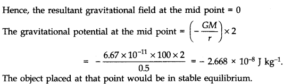## Question 8. 22. As you have learnt in the text, a geostationary satellite orbits the Earth at a height of nearly 36,000 km from the surface of the Earth. What is the potential due to Earth’s gravity at the site of this satellite? (Take the potential energy at infinity to be zero). Mass of the Earth = 6.0 x1024kg, radius = 6400 km.

### Answer:Distance of satellite from the centre of earth = R = r + x = 6400 + 36000 = 42400 km = 4.24 x 107m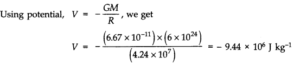## Question 8. 23. A star 2.5 times the mass of the sun and collapsed to a size of 12 km rotates with a speed of 1.2 rev. per second. (Extremely compact stars of this kind are known as neutron stars. Certain stellar objects called pulsars belong to this category). Will an object placed on its equator remain stuck to its surface due to gravity? (mass of the sun = 2 x 1030kg).

### Answer:Acceleration due to gravity of the star,g= GM/R2…………(i) Here M is the mass and R is the radius of the star. The outward centrifugal force acting on a body of mass m at the equator of the star =mv2/R =mR w2——-(ii) From equation (i), the acceleration due to the gravity of the star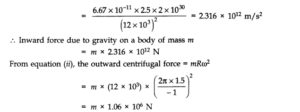## Question 8. 24. A spaceship is stationed on Mars. How much energy must be expended on the spaceship to rocket it out of the solar system? Mass of the spaceship = 1000 kg, Mass of the Sun = 2 x 1030kg. Mass of the Mars = 6.4 x 1023kg, Radius of Mars = 3395 km. Radius of the orbit of Mars = 2.28 x 1011m, G = 6.67 x 10-11N m2kg-2.

### Answer:Let R be the radius of orbit of Mars and R’ be the radius of the Mars. M be the mass of the Sun and M’ be the mass of Mars. If m is the mass of the space-ship, then Potential energy of space-ship due to gravitational attraction of the Sun = – GM m/R Potential energy of space-ship due to gravitational attraction of Mars = – G M’ m/R’ Since the K.E. of space ship is zero, therefore,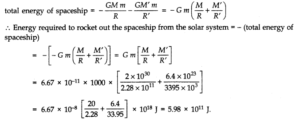## Question 8. 25. A rocket is fired ‘vertically’ from the surface of Mars with a speed of 2 km s-1. If 20% of its initial energy is lost due to Martian atmospheric resistance, how far will the rocket go from the surface of mars before returning to it? Mass of Mars = 6.4 x 1023kg; radius of Mars = 3395 km; G = 6.67 x 10-11N m2kg-2